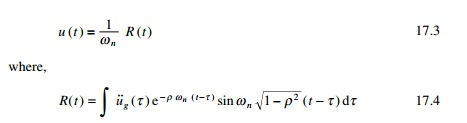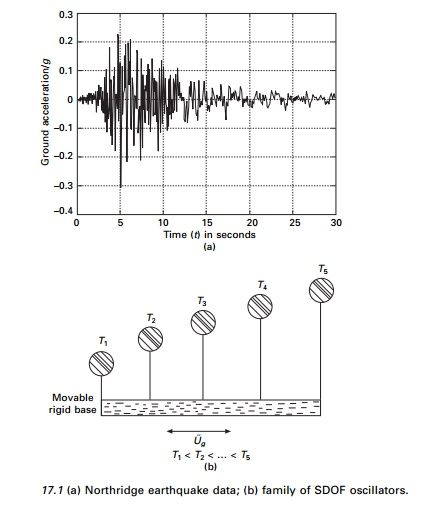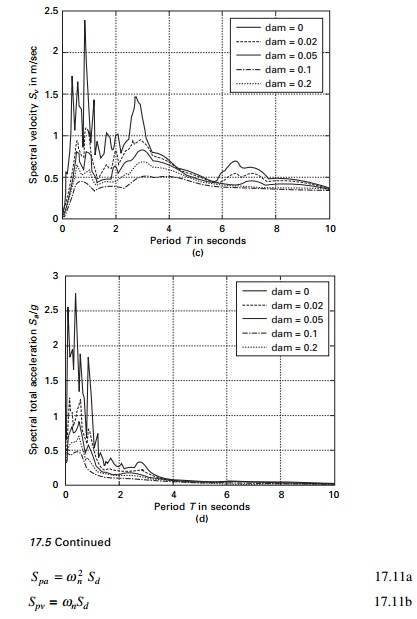Home | | Structural Dynamics and Earthquake Engineering | Earthquake response spectra

# Earthquake response spectra

Abstract: In this chapter, earthquake excitation problems are treated as base excitation problems. In many engineering applications, one requires maximum absolute quantities experienced by the structure during the earthquake of interest. In that respect, the response spectrum method is ideally suited to designing structures against earthquake motion. It is shown how the tripartite plot is useful for reading all the spectral quantities for a given period. The construction of a NewmarkŌĆōHall design spectrum is illustrated and the distinction is made between design and response spectra. In all the codes, site-specific response spectra are recommended. Finally inelastic design spectra are also discussed.

Earthquake response spectra

Abstract: In this chapter, earthquake excitation problems are treated as base excitation problems. In many engineering applications, one requires maximum absolute quantities experienced by the structure during the earthquake of interest. In that respect, the response spectrum method is ideally suited to designing structures against earthquake motion. It is shown how the tripartite plot is useful for reading all the spectral quantities for a given period. The construction of a NewmarkŌĆōHall design spectrum is illustrated and the distinction is made between design and response spectra. In all the codes, site-specific response spectra are recommended. Finally inelastic design spectra are also discussed.

Key words: accelerograph, response spectrum, spectral quantity, pseudo spectral quantity, ductility, time history.

Introduction

The earthquake response problem is essentially a base excitation problem similar to the one discussed in the earlier chapter for single-degree-of-freedom (SDOF) systems. The equation of motion is written asThus for any arbitrary acceleration of the supports the relative displacement of the mass can be computed using the Duhamel integral asFrom Eq. 17.2 it is seen that the relative response of the structure is characterized by its natural frequency, damping factor and the nature of base excitation.

Generally we use undamped natural frequency instead of damped frequency and the negative sign is ignored (the sense of response has no significance in earthquake analysis)v(t) = R(t) is called the earthquake response integral. The relative displacement is important since it is required to calculate base shear.

It is noticed that base shear represented in Eq. 17.5 is equivalent to restoring force Fs(t). The exact relative velocity is given by

The absolute total acceleration of the mass is obtained by adding relative acceleration with ground acceleration asThe total acceleration has many important applications. It can most easily be measured experimentally during a strong earthquake. When an accelerograph is located in a structure, it records in close approximation the total acceleration at that point. From this we can calculate inertia forceEquations 17.2, 17.6 and 17.7 represent the earthquake time history response for an SDOF system. Once we know base shear V (effective earthquake force), we can design a system.

Earthquake response spectra

It has been seen from earlier chapters that the evaluation of dynamic response (displacement, velocity and acceleration) at every instant of time during an earthquake requires significant computational effort even for relatively simple structural systems. However, for many engineering applications we require maximum absolute quantities experienced by the structure during the earthquake. These are commonly referred to as spectral displacement Sd, spectral velocity Sv and spectral acceleration Sa, given byare the maximum absolute values of  relative displacement, relative velocity and total acceleration determined from Eq. 17.2, 17.6 and 17.7. However, these quantities are generally determined from numerical integration techniques. Plots of Sd, Sv, Sa versus undamped natural period of vibration for various damping ratios are called earthquake response spectra. We can construct an earthquake response spectrum, say, for Northridge earthquake (see Fig 17.1a) by considering a series of oscillators (inverted pendulums) as shown in Fig. 17.1b with varying periods of vibration attached with movable base. The base is subjected to same ground motion as that of Northridge earthquake. The maximum response for each pendulum (Sd, Sv, Sa) is plotted against the natural period for a particular value of damping. Such curves are the response spectra and are very useful for design. Figure 17.2 shows response spectra for relative displacement, relative velocity and total acceleration for the Northridge earthquake.          These quantities are generally determined by numerical evaluation using any of the numerical techniques developed in Previous Pages. Plots of Sd, Sv and Sa versus undamped natural period of vibration or natural frequency for various damping factors make up the earthquake response spectrum. The MATLAB program for drawing the spectra in Fig. 17.2 using WilsonŌĆÖs recurrence formulae is shown below.

Program 17.1: MATLAB program for drawing spectra for any specified earthquake

%********************************************************** % WILSONŌĆÖS RECURRENCE FORMULA TO DRAW SPECTRA FOR ANY EARTHQUAKE MOTION %**********************************************************clc;close all;

%give initial displacement and initial velocity u(1)=0;

v(1)=0;

%tt=50 sec n=2500 for nridge %tt=30 n=1500 for elcentro ns tt=50.0;

n=2500;

n1=n+1;

dt=tt/n;

mass=1;

%**********************************************************

%      EARTHQUAKE DATA FILE FOR NORTH-RIDGE EQ IS READ FROM EXCEL DATA FILE

%      DATA FILE CONSISTS OF TIME AND THE CORRESPONDING A/G VALUES

%DATA FILE CHOPRA CONSISTS OF ELCENTRO NS DATA

EQDATA CONTAINS NORTHRIDGE DATA%**********************************************************

%de=xlsread(ŌĆśchopraŌĆÖ) for i=1:n1; ug(i)=de(i,2); ug(i)=-ug(i)*9.81; p(i)=ug(i)*mass;

end;

%define damping ratios for which response is required rho=[0 0.02 0.05 .1 0.2];

for jj=1:5 r=rho(jj);

mass=1; for ii=1:200

tn=0.05*ii;

wn=2.0*pi/tn;

ss(ii)=tn;

k=mass*wn^2;

c=2.0*r*sqrt(k*mass); wd=wn*sqrt(1-r^2);

a=exp(-r*wn*dt)*(r*sin(wd*dt)/sqrt(1-r^2)+cos(wd*dt)); b=exp(-r*wn*dt)*(sin(wd*dt))/wd; c2=((1-2*r^2)/(wd*dt)-r/sqrt(1-r^2))*sin(wd*dt)-(1+2*r/(wn*dt))*cos(wd*dt); c=(1/k)*(2*r/(wn*dt)+exp(-r*wn*dt)*(c2));

d 2 = e x p ( - r * w n * d t ) * ( ( 2 . 0 * r ^ 2 - 1 ) / ( w d * d t ) * s i n ( w d * d t ) + 2 . 0 * r / (wn*dt)*cos(wd*dt));

c 1 = e x p ( - r * w n * d t ) * ( ( w n / s q r t ( 1 - r ^ 2 ) + r / ( d t * s q r t ( 1 - r^2)))*sin(wd*dt)+cos(wd*dt)/dt);

cd=(1/k)*(-1/dt+c1); d1=exp(-r*wn*dt)*(r*sin(wd*dt)/sqrt(1-r^2)+cos(wd*dt)); dd=(1/(k*dt))*(1-d1);

end dmf(ii,jj)=max(abs(u)); vmf(ii,jj)=max(abs(v)); amf(ii,jj)=max(abs(ta)); end

end

for m=1:n1 s(m)=(m-1)*dt;

end figure(1);

plot(s,ug/9.81,ŌĆśKŌĆÖ);

xlabel(ŌĆś time (t) in secondsŌĆÖ); ylabel(ŌĆś ground acceleration/gŌĆÖ); title(ŌĆś North Ridge EQ dataŌĆÖ); figure(2);

for ii=1:5 plot(ss,dmf(:,ii),ŌĆśKŌĆÖ); hold on

end

xlabel(ŌĆś period T in secondsŌĆÖ);

ylabel(ŌĆś Spectral displacement Sd in mŌĆÖ);

title(ŌĆś Response spectrum for Sd North Ridge EQ)ŌĆÖ);

legend(ŌĆś dam=0ŌĆÖ,ŌĆśdam=0.02ŌĆÖ,ŌĆśdam=0.05ŌĆÖ, ŌĆśdam=0.1ŌĆÖ,ŌĆśdam=0.2ŌĆÖ) figure(3);

for ii=1:5 plot(ss,vmf(:,ii),ŌĆśKŌĆÖ); hold on

end

xlabel(ŌĆś period T in secondsŌĆÖ);

ylabel(ŌĆś Spectral velocity Sv in m/secŌĆÖ);

title(ŌĆś Response spectrum for Sv (North Ridge EQ)ŌĆÖ);

legend(ŌĆś dam=0ŌĆÖ,ŌĆśdam=0.02ŌĆÖ,ŌĆśdam=0.05ŌĆÖ, ŌĆśdam=0.1ŌĆÖ,ŌĆśdam=0.2ŌĆÖ) figure(4);

for ii=1:5 plot(ss,amf(:,ii)/9.81,ŌĆśKŌĆÖ); hold on

end

xlabel(ŌĆś period T in secondsŌĆÖ);

ylabel(ŌĆś Spectral total acceleration Sa/gŌĆÖ);

title(ŌĆś Response spectrum for Sa (total) (North Ridge EQ)ŌĆÖ); legend(ŌĆś dam=0ŌĆÖ,ŌĆśdam=0.02ŌĆÖ,ŌĆśdam=0.05ŌĆÖ, ŌĆśdam=0.1ŌĆÖ,ŌĆśdam=0.2ŌĆÖ) fid=fopen(ŌĆśsv.outŌĆÖ,ŌĆśwŌĆÖ)

for jj=1:200

fprintf(fid,ŌĆś %6.3f %6.2f %6.2f %6.2f %6.2f %6.2f\nŌĆÖ...

,ss(jj),vmf(jj,1),vmf(jj,2),vmf(jj,3),vmf(jj,4),vmf(jj,5)); end

fclose(fid)

Example 17.1

An industrial building is shown in Fig. 17.3. Idealize the structure as an SDOF system. Assume the structure acts as a braced frame in the EW direction (having a total of six braced bays) and unbraced shear frame (with column base) pinned in the NS direction. Assume all columns bend about the major axis to the NS direction.

The vertical cross-bracings in the EW direction are 25.4 mm diameter steel rods. The dead weight of the structure is 1291.95 kN which is concentrated at the base of the roof trusses. Moment of inertia of columns 8.6992 ├Ś 107 mm4. The height of the building may be assumed as 4.2672 m and the damping is 5% of critical damping. Consider Northridge earthquake.

(a)  Determine the natural period for the NS and EW directions.

(b) Conduct a time history analysis of the structure in both directions. Use the NS component of the 17 January, 1994 Northridge earthquake shown in Fig. 17.1a as input.

Solution

(a)  Natural period. Mass of the structure(b) Time history response. The parameters in the time history response are relative displacement, u(t), relative velocity is u╦Ö(t ), absolute total acceleration is u╦Ö╦Öt (t ). Also of interest of base shear V(t), the bending moment in columns M(t) and axial force in the steel rods T(t).

The equation of moment in NS directionEquation of moment in EW direction

u╦Ö╦Ö + 2 ├Ś 0.05 ├Ś 20 u╦Ö + 20 2 u = ŌłÆu╦Ö╦Ög (t )

i.e. u╦Ö╦Ö + 2 u╦Ö + 400 u = ŌłÆu╦Ö╦Ög (t )

The value can be obtained for the Northridge earthquake by integrating above two expressions.

As an example. The response displacement, velocity and total acceleration are shown in Fig. 17.4 and Table 17.1 gives maximum response in NS direction.Example 17.2

Construct response spectra for the NS component of E1 Centro earthquake (see Fig. 17.5a). Consider damping factors 0, 0.02, 0.05, 0.1 and 0.2.

Solution

The spectral displacement Sd, spectral velocity Sv and spectral acceleration Sa are determined from Eq. 17.9a, b and c respectively. The responses are evaluated numerically by direct integration of the equationfor Žü = 0.02, 0.05 and 0.1 from which maximum responses are determined. The response spectral Sd, Sv and Sa are presented in Fig. 17.5b, c and d due to the El Centro earthquake (NS) shown in Fig. 17.5a.

Let us define pseudo-spectral velocity and pseudo spectral acceleration as Spv, Spa as Spa = Žē n2 Sd = Žēn Sv; Spv = Žēn Sd. Usually the parameters Spv and Spa have certain characteristics that are of practical interest. The pseudo-spectral velocity Spv is close to spectral velocity Sv for short period structure. The comparison between Spv and Sv for the NS component of the El Centro earthquake for Žé = 0.05 is illustrated in Fig. 17.6. For zero damping, the pseudo-spectral acceleration Spa is identical to spectral acceleration. However, for damping other than zero, the two are slightly different. Nevertheless for damping levels encountered in most engineering applications, the two can be considered practically equal.Hence the spectral relationship significantly expedites the construction of earthquake response spectra. Evaluation of spectra displacement sd after numerical integration to obtain time history response, the corresponding pseudo spectral velocity Spv and pseudo spectral acceleration Spa can readily be established and we see later how sd, spv and Spa can then all be plotted on a four-way logarithmic paper.

Then for a given frequency or for a given period all the spectral quantities can be read simultaneously for the same tripartite plot. A tripartite plot of sd,

spvr, Spa for the NS component of the El Centro earthquake for various damping factors is presented in Fig. 17.7.Program 17.2: MATLAB program to draw tripartite plot

%**********************************************************

% to draw elastic design spectra from the earthquake data

% read the response spectrum values in this program

%**********************************************************

for k=.00001:.00001:.0001

x=0.01:1:100

t=log(2*pi*k)-log(x)

y=exp(t)

loglog(x,y,ŌĆśkŌĆÖ),grid on

hold on

t=log(k*9.81/(2*pi))+log(x)

y=exp(t)

loglog(x,y,ŌĆśkŌĆÖ)

hold on

end

for k=.0001:.0001:.001

x=0.01:1:100

t=log(2*pi*k)-log(x)

y=exp(t)

loglog(x,y,ŌĆśkŌĆÖ),grid on

hold on

t=log(k*9.81/(2*pi))+log(x)

y=exp(t)

loglog(x,y,ŌĆśkŌĆÖ) hold on

end

for k=.001:.001:.01 x=0.01:1:100 t=log(2*pi*k)-log(x) y=exp(t) loglog(x,y,ŌĆśkŌĆÖ),grid on hold on

t=log(k*9.81/(2*pi))+log(x)

y=exp(t)

loglog(x,y,ŌĆśkŌĆÖ) hold on

end

xlabel(ŌĆś period in secsŌĆÖ)

ylabel(ŌĆś spectral velocity sv in cm/secŌĆÖ) for k=.01:.01:.1

x=0.01:1:100 t=log(2*pi*k)-log(x) y=exp(t) loglog(x,y,ŌĆśkŌĆÖ),grid on hold on

t=log(k*9.81/(2*pi))+log(x)

y=exp(t)

loglog(x,y,ŌĆśkŌĆÖ) hold on

end

for k=.1:.1:1 x=0.01:1:100 t=log(2*pi*k)-log(x) y=exp(t) loglog(x,y,ŌĆśkŌĆÖ),grid on hold on

t=log(k*9.81/(2*pi))+log(x)

y=exp(t)

loglog(x,y,ŌĆśkŌĆÖ) hold on

end

for k=1:1:10 x=0.01:1:100 t=log(2*pi*k)-log(x) y=exp(t)

loglog(x,y,ŌĆśkŌĆÖ),grid on hold on

t=log(k*9.81/(2*pi))+log(x)

y=exp(t)

loglog(x,y,ŌĆśkŌĆÖ) hold on

end

for k=10:10:100 x=0.01:1:100 t=log(2*pi*k)-log(x) y=exp(t) loglog(x,y,ŌĆśkŌĆÖ),grid on hold on

t=log(k*9.81/(2*pi))+log(x)

y=exp(t)

loglog(x,y,ŌĆśkŌĆÖ) hold on

end

for k=100:100:1000 x=0.01:1:100 t=log(2*pi*k)-log(x) y=exp(t) loglog(x,y,ŌĆśkŌĆÖ),grid on hold on

t=log(k*9.81/(2*pi))+log(x)

y=exp(t)

loglog(x,y,ŌĆśkŌĆÖ) hold on

end

for k=1000:1000:10000 x=0.01:1:100 t=log(2*pi*k)-log(x) y=exp(t) loglog(x,y,ŌĆśkŌĆÖ),grid on hold on

t=log(k*9.81/(2*pi))+log(x)

y=exp(t)

loglog(x,y,ŌĆśkŌĆÖ) end

axis([0.01 100 0.02 500]) % d=xlsread(ŌĆśsvdataŌĆÖ); sv=ŌĆśsv.outŌĆÖ

plot(d(:,1),100*d(:,2),ŌĆśkŌĆÖ)

plot(d(:,1),100*d(:,3),ŌĆśkŌĆÖ)

plot(d(:,1),100*d(:,4),ŌĆśkŌĆÖ)

plot(d(:,1),100*d(:,5),ŌĆśkŌĆÖ)

plot(d(:,1),100*d(:,6),ŌĆśkŌĆÖ)

text(0.2,0.02,ŌĆś0.001ŌĆÖ);

text(0.6,0.1,ŌĆś0.01ŌĆÖ);

text(2,0.3,ŌĆś0.1ŌĆÖ);

text(7,1,ŌĆś1ŌĆÖ);

text(20,3,ŌĆś10ŌĆÖ);

text(80,10,ŌĆś100ŌĆÖ) text(20,1,ŌĆśsd in cmŌĆÖ) xlabel(ŌĆś period in secŌĆÖ) ylabel(ŌĆś sv in cm/secŌĆÖ) text(0.01,200,ŌĆś100ŌĆÖ) text(0.01,20,ŌĆś10ŌĆÖ) text(0.01,2,ŌĆś1ŌĆÖ) text(0.02,0.4,ŌĆś0.1ŌĆÖ) text(0.07,0.1,ŌĆś0.01ŌĆÖ) text(.02,0.8,ŌĆÖsa/gŌĆÖ) gtext(ŌĆś no dampingŌĆÖ) gtext(ŌĆś damping=2%ŌĆÖ) gtext(ŌĆś damping=5%ŌĆÖ) gtext(ŌĆś damping=10%ŌĆÖ) gtext(ŌĆś damping=20%ŌĆÖ)

Study Material, Lecturing Notes, Assignment, Reference, Wiki description explanation, brief detail
Civil : Structural dynamics of earthquake engineering : Earthquake response spectra |